# What Is the Potential Difference Across Each Capacitor?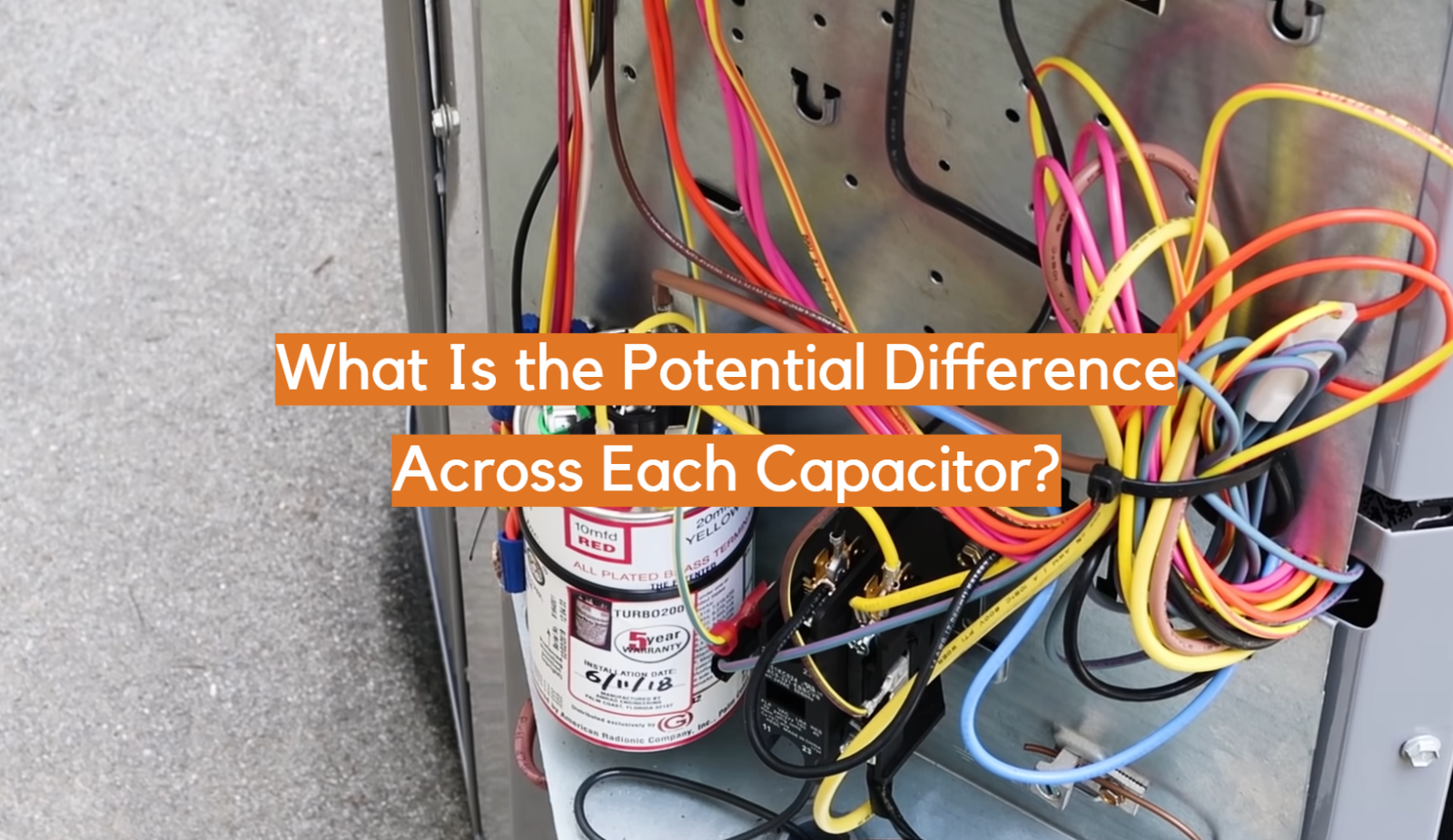Capacitors are fundamental components in electrical circuits that store and release electrical energy. When analyzing capacitor circuits, one important aspect to consider is the potential difference across each capacitor. The potential difference, also known as voltage, determines the energy stored in the capacitor and affects its behavior within the circuit. In this article, we will explore how to calculate the potential difference across capacitors in different circuit configurations.

## What Is the Potential Difference in Capacitors?

The potential difference, also known as voltage, is a fundamental concept in electrical circuits, and it plays a crucial role in understanding the behavior of capacitors. Capacitors are passive electronic components that store and release electrical energy, and the potential difference across them directly influences their performance.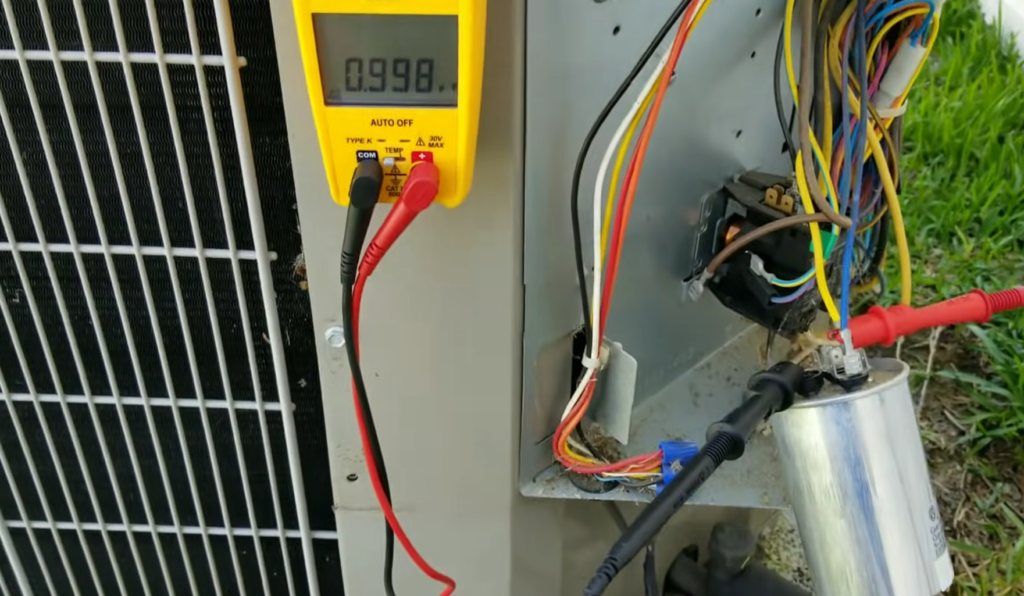In simple terms, the potential difference in capacitors refers to the voltage across the plates of the capacitor. A capacitor consists of two conductive plates separated by a dielectric material. When a potential difference is applied across the plates, an electric field is established, causing positive and negative charges to accumulate on the respective plates.

The potential difference in capacitors determines the amount of electric potential energy stored in the capacitor. It represents the work done to move a unit charge from one plate to the other and is measured in volts (V) .

In a circuit with capacitors, the potential difference can vary depending on the circuit configuration. Let’s consider two common configurations: capacitors in series and capacitors in parallel.

When capacitors are connected in series, they share the same current but have different voltage drops across them. The total potential difference across the series combination of capacitors is equal to the sum of the individual voltage drops. Each capacitor in the series has a different potential difference, and the total potential difference is divided among them according to their capacitance values.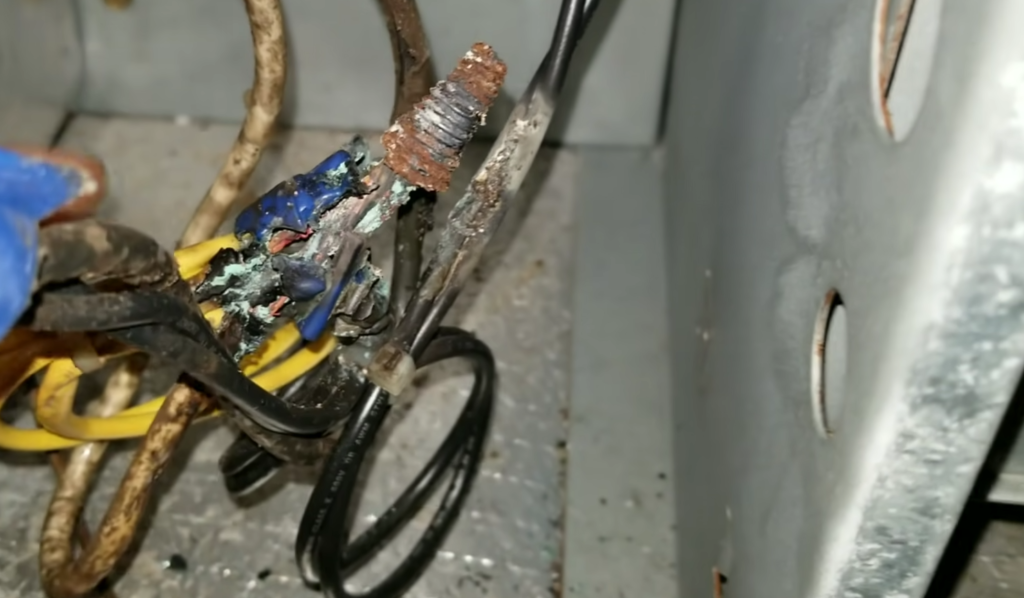On the other hand, when capacitors are connected in parallel, the potential difference across each capacitor is the same and equal to the applied voltage. In a parallel configuration, the voltage is shared across all the capacitors, and each capacitor experiences the full potential difference.

To calculate the potential difference in capacitors, it is necessary to analyze the circuit configuration and apply the appropriate formulas. In series configurations, you need to calculate the equivalent capacitance of the series combination and then determine the potential difference across each capacitor using the ratio of the individual capacitance to the equivalent capacitance.

In parallel configurations, the potential difference is the same across all capacitors and equal to the applied voltage.

Understanding the potential difference in capacitors is essential for designing and analyzing electrical circuits. It enables engineers and technicians to accurately determine the voltage requirements and energy storage capabilities of capacitors in various applications, including power supplies, filtering circuits, timing circuits, and energy storage systems. By harnessing the potential difference effectively, capacitors contribute to the efficient and reliable operation of electronic devices and systems.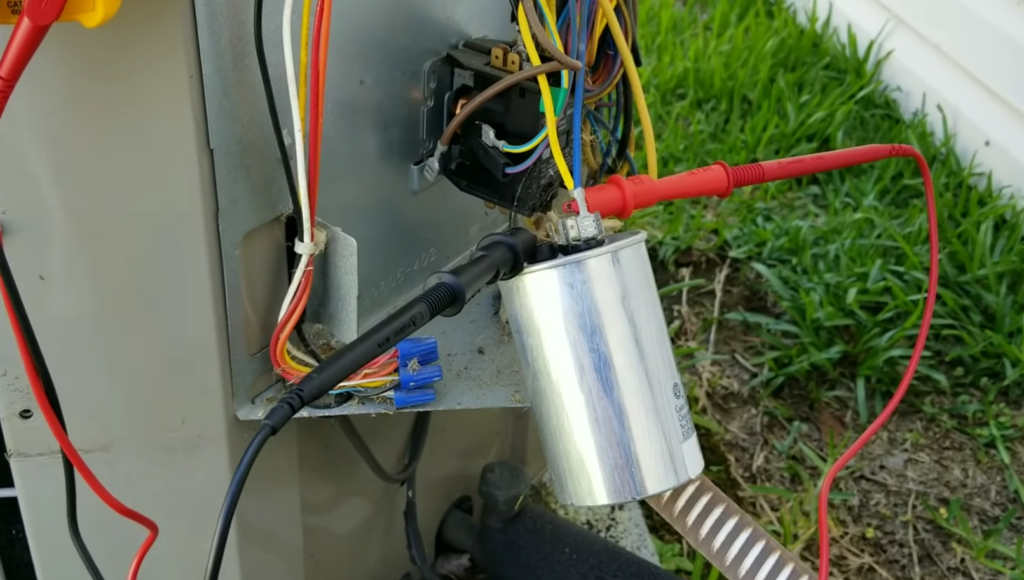### What Is the Potential Difference Across Each Capacitor?

In a circuit with multiple capacitors, the potential difference across each capacitor can vary depending on the circuit configuration. There are two common configurations to consider: capacitors in series and capacitors in parallel. Let’s explore each of these scenarios in detail.

### How Do You Find the Potential Difference Across Each Capacitor: Capacitors in a Series Connection

When capacitors are connected in series, they share the same current but have different voltage drops across them. To find the potential difference across each capacitor in a series connection, we need to understand the concept of equivalent capacitance.

The equivalent capacitance (Ceq) for capacitors in series can be calculated using the formula:

1/Ceq = 1/C1 + 1/C2 + 1/C3 + … (for n capacitors in series)

Once we have the equivalent capacitance, we can determine the potential difference across each capacitor. The voltage across each capacitor can be found using the formula:

• V1 = (Ceq / C1) * Vs
• V2 = (Ceq / C2) * Vs
• V3 = (Ceq / C3) * Vs

where Vs is the total voltage applied across the series capacitors.

### How Do You Find the Potential Difference Across Each Capacitor: Capacitors in a Parallel Connection

In a parallel configuration, the potential difference across each capacitor is the same. The total potential difference across the parallel capacitors is equal to the applied voltage.

To find the potential difference across each capacitor in a parallel connection, there is no need to calculate an equivalent capacitance. The voltage across each capacitor is simply equal to the total applied voltage:

V1 = V2 = V3 = … = Vn = Vp

where Vp is the applied voltage across the parallel capacitors.

### The Formula for Voltage Drop Across Capacitor: Voltage Across an Uncharged Capacitor

In addition to the potential difference across capacitors in different circuit configurations, it is important to understand the voltage behavior during different stages of capacitor operation. Let’s consider the voltage drop across a capacitor during various situations.

When an uncharged capacitor is connected to a circuit, initially, the potential difference across it is zero. As the capacitor charges up, the voltage across the capacitor gradually increases until it reaches the same value as the applied voltage.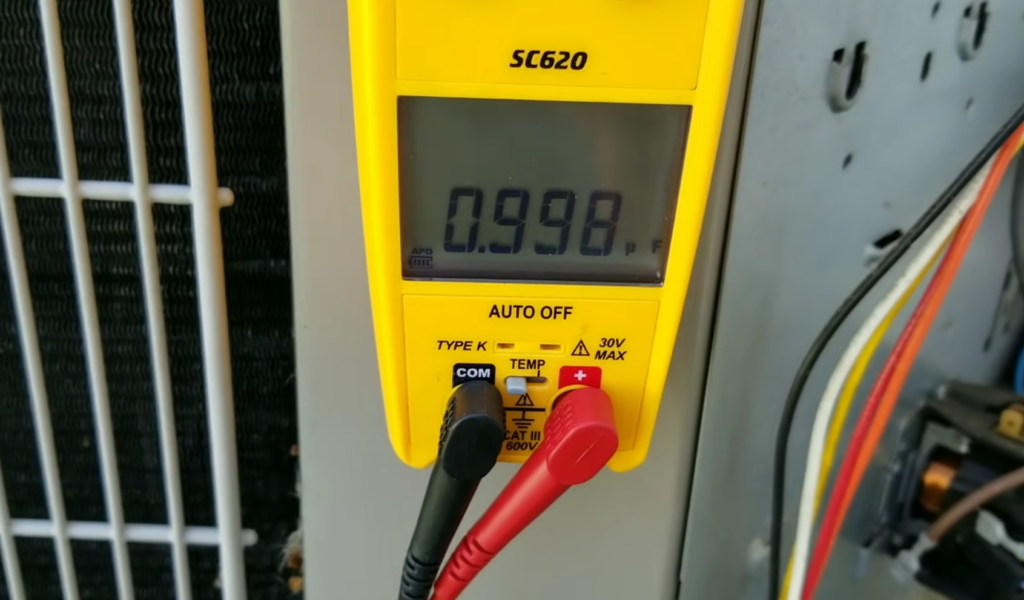The voltage across an uncharged capacitor can be calculated using the formula:

Vc(t) = Vs * (1 – e^(-t / (R * C)))

where Vc(t) represents the voltage across the capacitor at a specific time t, Vs is the applied voltage, R is the resistance in the circuit, and C is the capacitance of the capacitor .

### Voltage Across a Capacitor During Charging

During the charging process, the potential difference across the capacitor increases. The rate at which the voltage increases depends on the time constant of the circuit, which is determined by the product of resistance and capacitance (R * C). The voltage across a capacitor during charging can be calculated using the same formula as above.

### Voltage Drop Across a Completely Charged Capacitor

Once a capacitor is completely charged, the potential difference across it remains constant and equal to the applied voltage. At this stage, the capacitor acts as an open circuit, and no current flows through it.

### Voltage Across Capacitor During Discharging

When a charged capacitor is discharged, the potential difference across it gradually decreases. The voltage drop can be calculated using the formula:

Vc(t) = Vs * e^(-t / (R * C))

where Vc(t) represents the voltage across the capacitor at a specific time t during the discharging process.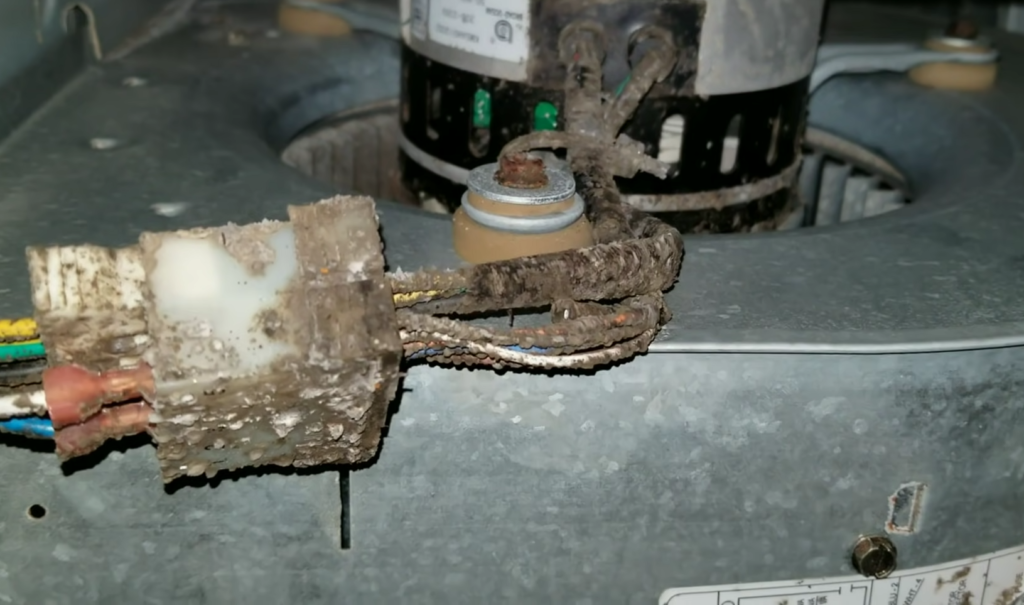## FAQ:

### 1. How do you find the potential difference across each capacitor?

The potential difference across each capacitor can be found by analyzing the circuit configuration and using appropriate formulas. For capacitors in series, you need to calculate the equivalent capacitance (Ceq) using the formula: 1/Ceq = 1/C1 + 1/C2 + 1/C3 +.

Once you have Ceq, you can determine the potential difference across each capacitor using the formula: V1 = (Ceq / C1) * Vs, V2 = (Ceq / C2) * Vs, V3 = (Ceq / C3) * Vs, where Vs is the total applied voltage .

In the case of capacitors in parallel, the potential difference across each capacitor is the same and equal to the applied voltage. Therefore, you don’t need to calculate an equivalent capacitance. The voltage across each capacitor is simply equal to the total applied voltage.

### 2. What is the potential difference across a charged capacitor?

The potential difference across a charged capacitor depends on the amount of charge stored in the capacitor and is equal to the voltage applied to the capacitor.

When a capacitor is fully charged, the potential difference across it remains constant and equal to the applied voltage.

### 3. Is the potential difference across capacitors the same?

The potential difference across capacitors can vary depending on the circuit configuration. In capacitors connected in series, each capacitor has a different potential difference. However, in capacitors connected in parallel, the potential difference across each capacitor is the same and equal to the applied voltage.

### 4. What is the voltage difference across the capacitor?

The voltage difference across a capacitor refers to the potential difference between its two plates. It represents the electric potential energy stored in the capacitor and determines its ability to store and release electrical charge.

### 5. What is the voltage potential across the capacitor?

The voltage potential across a capacitor is the same as the potential difference across the capacitor. It signifies the voltage between the positive and negative plates of the capacitor.

### 6. What is the potential of a capacitor?

The potential of a capacitor refers to the potential difference or voltage across its terminals. It represents the electric potential energy per unit charge stored in the capacitor.

### 7. What is the potential difference across two capacitors in a circuit?

The potential difference across two capacitors in a circuit depends on the circuit configuration. If the capacitors are in series, each capacitor will have a different potential difference. If the capacitors are in parallel, the potential difference across each capacitor will be the same.

### 8. What is the potential difference across two capacitors in series?

When capacitors are connected in series, the potential difference across each capacitor depends on the ratio of its capacitance to the total equivalent capacitance of the series combination. The potential difference across each capacitor is determined using the formula: V1 = (Ceq / C1) * Vs, V2 = (Ceq / C2) * Vs, V3 = (Ceq / C3) * Vs, where Ceq is the equivalent capacitance and Vs is the total applied voltage.

### 9. How do you calculate potential differences?

To calculate potential differences, you need to know the circuit configuration and apply the appropriate formulas. For capacitors in series, you calculate the equivalent capacitance and use it to find the potential difference across each capacitor. For capacitors in parallel, the potential difference across each capacitor is equal to the applied voltage.

### 10. What is the potential difference across capacitor C3?

To determine the potential difference across capacitor C3, you need more specific information about the circuit configuration and the voltages applied. Depending on the circuit, the potential difference across C3 can be calculated using the appropriate formulas for series or parallel capacitors.

### 11. Is potential difference the same as voltage?

Yes, potential difference and voltage are used interchangeably. Potential difference refers to the difference in electric potential between two points in a circuit, while voltage represents the magnitude of that potential difference.

### 12. What is the potential difference across capacitors in an LCR circuit?

In an LCR (inductor-capacitor-resistor) circuit, the potential difference across the capacitors will depend on the specific configuration and the voltages applied. Without further details, it is not possible to provide a specific answer.

### 13. What is the formula for the potential of a capacitor?

The potential of a capacitor is not typically expressed using a specific formula. Instead, the potential difference across a capacitor is often discussed, which represents the voltage between its plates and can be calculated using appropriate formulas for different circuit configurations.

### 14. What is the common potential between two capacitors?

In general, the common potential between two capacitors depends on the circuit configuration and the voltages applied. If the capacitors are connected in parallel, they will have the same potential difference or voltage. However, if they are connected in series, each capacitor will have a different potential difference.

### 15. What is the formula for the charge across each capacitor?

The formula for calculating the charge across a capacitor depends on the capacitance (C) and the potential difference (V) across it. The charge (Q) can be calculated using the formula: Q = C * V.

### 16. What is an example of a potential difference?

An example of a potential difference is the voltage across a battery. When a battery is connected to a circuit, there is a potential difference between its positive and negative terminals, which creates an electric field and drives the flow of current.

### 17. What is the value of the potential difference?

The value of the potential difference depends on the specific circuit and the voltages applied. It can vary widely, from millivolts to kilovolts or even higher, depending on the electrical system and its requirements.

### 18. Why is the potential difference the same in parallel?

The potential difference is the same in parallel because, in a parallel configuration, all components are connected across the same two points. Therefore, the voltage or potential difference across each component in parallel is equal to the potential difference between the points where the components are connected.

### 19. What is the potential difference across the 12 Ω resistor?

To determine the potential difference across a specific resistor, you need additional information, such as the current flowing through the resistor or the total voltage applied to the circuit. Using Ohm’s Law (V = I * R), you can calculate the potential difference across the 12 Ω resistor if you know the current passing through it.

### 20. What is the potential difference across the 4.5 µF capacitor?

To determine the potential difference across a capacitor, you need additional information about the circuit configuration and the voltages applied. Without specific details, it is not possible to provide a precise answer.

### 21. Does potential drop across a capacitor?

Yes, there can be a potential drop across a capacitor, especially when it is connected in a circuit with other components. The potential drop across a capacitor occurs when there is a voltage difference between its two plates due to the charge stored on them.

### 22. How do you find the potential energy of a capacitor?

The potential energy stored in a capacitor can be calculated using the formula: U = (1/2) * C * V^2, where U represents the potential energy, C is the capacitance of the capacitor, and V is the potential difference or voltage across it.